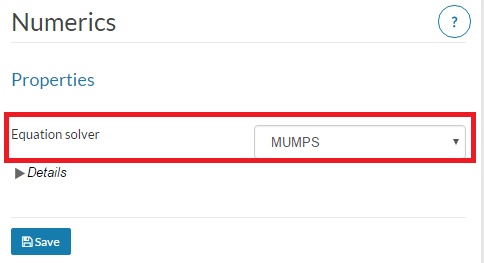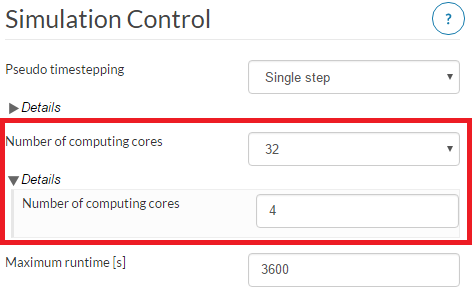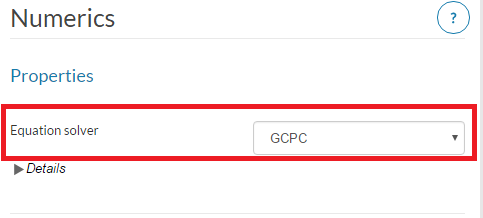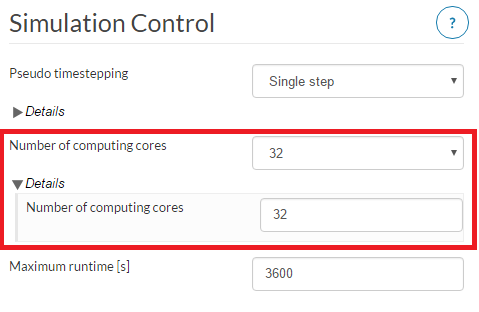# Choosing a solver for 2nd order mesh

A potential issue with using a 2nd order mesh is memory allocation where the mesh may be too large for the machine size and solver that have been chosen (default = Multfront).

To tackle this there are a couple of options depending on the equation solver that you want to use:

1. Direct Solver

If you want to use a direct solver, MUMPS will be a better choice. You will find this in the project tree under Numerics.In Simulation Control, set the Number of computing cores to be 32 and in Details set the Number of computing cores (parallel) to be 2 - 4.2. Iterative Solvers

An iterative solver could also be an option to reduce the memory requirements. For this, choose the equation solver in Numerics to be GCPC or PETSC.In Simulation Control for GCPC, set the Number of computing cores to be 32 and in Details the Number of computing cores (parallel) can also be 32 for this solver.For PETSC, set the Number of computing cores to be 32 and in Details set the Number of computing cores (parallel) to 4-8.

3 Likes